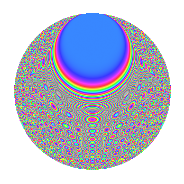# Properties

 Label 1512.2.ddLevel 1512 Weight 2 Character orbit dd Rep. character $$\chi_{1512}(193,\cdot)$$ Character field $$\Q(\zeta_{9})$$ Dimension 432 Sturm bound 576

# Related objects

## Defining parameters

 Level: $$N$$ = $$1512 = 2^{3} \cdot 3^{3} \cdot 7$$ Weight: $$k$$ = $$2$$ Character orbit: $$[\chi]$$ = 1512.dd (of order $$9$$ and degree $$6$$) Character conductor: $$\operatorname{cond}(\chi)$$ = $$189$$ Character field: $$\Q(\zeta_{9})$$ Sturm bound: $$576$$

## Dimensions

The following table gives the dimensions of various subspaces of $$M_{2}(1512, [\chi])$$.

Total New Old
Modular forms 1776 432 1344
Cusp forms 1680 432 1248
Eisenstein series 96 0 96

## Trace form

 $$432q + O(q^{10})$$ $$432q + 12q^{15} + 24q^{17} + 12q^{21} - 24q^{23} + 18q^{29} - 36q^{39} + 12q^{41} + 18q^{45} - 18q^{47} - 36q^{49} - 18q^{61} + 18q^{63} + 72q^{65} + 48q^{69} - 72q^{75} - 84q^{77} + 72q^{81} + 72q^{89} + 72q^{93} + 54q^{95} - 108q^{99} + O(q^{100})$$

## Decomposition of $$S_{2}^{\mathrm{new}}(1512, [\chi])$$ into newform subspaces

The newforms in this space have not yet been added to the LMFDB.

## Decomposition of $$S_{2}^{\mathrm{old}}(1512, [\chi])$$ into lower level spaces

$$S_{2}^{\mathrm{old}}(1512, [\chi]) \cong$$ $$S_{2}^{\mathrm{new}}(189, [\chi])$$$$^{\oplus 4}$$$$\oplus$$$$S_{2}^{\mathrm{new}}(378, [\chi])$$$$^{\oplus 3}$$$$\oplus$$$$S_{2}^{\mathrm{new}}(756, [\chi])$$$$^{\oplus 2}$$

## Hecke Characteristic Polynomials

There are no characteristic polynomials of Hecke operators in the database